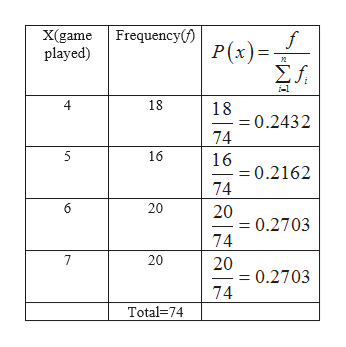# The following data represent the number of games played in each series of an annual tournament from 1928 to 2002. Complete parts (a) through (d) below.x (games played)Frequency46518716 20 20(a) Construct a discrete probability distribution for the random variable xx (games played)P(x)4567(Round to four decimal places as needed.)(b) Graph the discrete probability distribution. Choose the correct graph below.D.C.B.O A.APX)0.54054AP(x)054AP(x)0 54Click to select your answer(s).Type here to searchSUSEXAMP17t615EaCOf3f25

Questionhelp_outlineImage TranscriptioncloseThe following data represent the number of games played in each series of an annual tournament from 1928 to 2002. Complete parts (a) through (d) below. x (games played) Frequency 4 6 5 18 7 16 20 20 (a) Construct a discrete probability distribution for the random variable x x (games played) P(x) 4 5 6 7 (Round to four decimal places as needed.) (b) Graph the discrete probability distribution. Choose the correct graph below. D. C. B. O A. APX) 0.54 054 AP(x) 054 AP(x) 0 54 Click to select your answer(s). Type here to search SUS EXAMP 17 t6 15 Ea CO f3 f2 5 fullscreen
check_circle

Step 1

From the given information:

 X(game played) 4 5 6 7 Frequency 18 16 20 20

a.

Construct a discrete probability distribution for the random variable x as follows:

...help_outlineImage TranscriptioncloseX(game played) Frequency(f) f P(x) Σ. 2 4 18 18 =0.2432 74 5 16 16 0.2162 74 6 20 20 =0.2703 74 7 20 20 =0.2703 74 Total=74 fullscreen

### Want to see the full answer?

See Solution

#### Want to see this answer and more?

Solutions are written by subject experts who are available 24/7. Questions are typically answered within 1 hour.*

See Solution
*Response times may vary by subject and question.
Tagged in

### Statistics